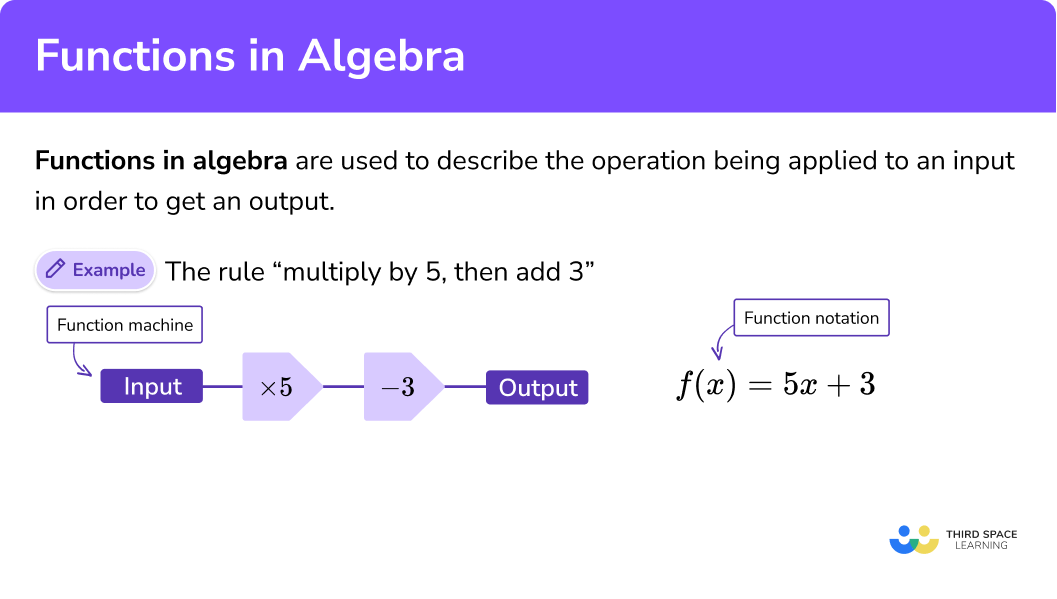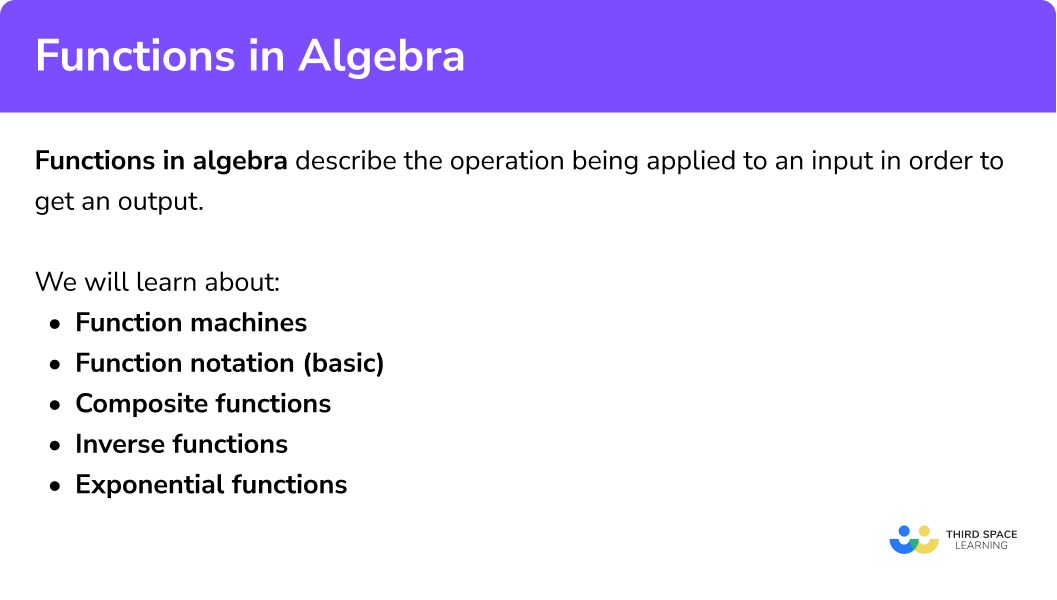GCSE Maths Algebra

Functions

# Functions In Algebra

Here we will learn about functions in algebra, including function machines, composite functions and inverse functions.

There are also functions worksheets based on Edexcel, AQA and OCR exam questions, along with further guidance on where to go next if you’re still stuck.

## What are functionsin algebra?

Functions in algebra are used to describe the operation being applied to an input in order to get an output.

### What are functions in algebra?### Function machines

Functions can be presented using a function machine.

Step-by-step guide: Function machines

### Function notation

Functions can be described using function notation.

The f in f(x) is known as the function that being applied to a variable x . Other letters such as g and h are also commonly used.

E.g.

Let’s look at a function f described by a function machine:

Then let’s rewrite this using function notation.

Step-by-step guide: Function notation

### Inverse and composite functions

Functions can be extended to work with inverse functions and composite functions. Inverse functions ‘reverse’ or ‘undo’ the original function. Composite functions combine two or more functions.

### Composite functions

We can combine functions to make composite functions.

Step-by-step guide: Composite functions

### Inverse functions

We can also find inverse functions.

Step-by-step guide: Inverse functions

### How to solve an equation using a function## How to use function machines

In order to solve an equation using a function machine:

1. Consider the order of operations being applied to the unknown.
2. Draw a function machine starting with the unknown as the input and the value of the equation as the output.
3. Work backwards, applying inverse operations to find the unknown input.

## Function machines example

### Example 1: solving a two step equation using a function machine

Solve \frac{x}{3}+7=3 .

1. Consider the order of operations being applied to the unknown.

The order of operations is \div 3 , then +7 .

2Draw a function machine starting with the unknown as the input and the value of the equation as the output.

3Work backwards, applying inverse operations to find the unknown input.

x=\left( 3-7 \right)\times 3

x=-12

## How to use function notation

In order to evaluate a function using function notation:

1. Write out the function for x using function notation, replacing the x with an empty set of brackets (parentheses).
2. Replace the x in the function with the number or algebraic term in the brackets next to the name of the function.
3. Apply the correct operations to the number or term as appropriate and simplify.

## Function notation examples

### Example 2: evaluating functions for numerical values

Find f(2) when f(x) = 3x + 2 .

Write out the function for x using function notation, replacing the x with an empty set of brackets.

Replace the x in the function with the number or algebraic term in the brackets next to the “name” of the function.

Apply the correct operations to the number or term as appropriate and simplify.

### Example 3: evaluating functions for algebraic expressions

Find f(4m) when f(x) = 5x − 7 .

Write out the function for x using function notation, replacing the x with an empty set of brackets.

Replace the x in the function with the number or algebraic term in the brackets next to the “name” of the function.

Apply the correct operations to the number or term as appropriate and simplify.

## How to evaluate composite functions

In order to evaluate composite functions:

1. Use the number to be evaluated as the input for the inner function and substitute it into the expression.
2. Find the output for the inner function and substitute it into the expression for the outer function.
3. Find the output for the outer function and repeat the process if there are any further outer functions.

## Composite functions example

### Example 4: evaluating composite functions

If f(x) = 3x and g(x) = x^2 + 1 , find fg(2) .

Use the number to be evaluated as the input for the inner function and substitute it into the expression.

Find the output for the inner function and substitute it into the expression for the outer function.

Find the output for the outer function and repeat the process if there are any further outer functions.

## How to find composite functions

In order to find composite functions:

1. Take the most inner function and substitute it into the next outer function wherever there is an x .
2. Simplify the expression as appropriate.
3. Repeat for any further outer functions.

## Composite function example

### Example 5: finding composite functions

If f(x) = 2x - 1 and g(x) = x^2 + 4 , find fg(x) .

Take the most inner function and substitute it into the next outer function wherever there is an x .

Simplify the expression as appropriate.

Repeat for any further outer functions.

## How to find inverse functions

In order to find an inverse function.

1. Write out the expression for the original function using a y instead of the x . Set this expression equal to x .
2. Rearrange the equation to make y the subject.
3. Write your inverse function using the f^{-1} notation.

## Inverse function examples

### Example 6: finding the inverse of a linear function

If f(x) = 3x + 7 , find f^{-1}(x) .

Write out the expression for the original function using a y instead of the x . Set this expression equal to x .

Rearrange the equation to make y the subject.

Write your inverse function using the f^{-1} notation.

### Common misconceptions

• Not working backwards when using inverse functions

When using function machines that include multiple operations to solve equations, a common error is to forget to work backwards. This means that the inverse operations are used but they are in the wrong order.

• Not using the correct order of operations when drawing a function machine

A common error is to not follow the correct order of operations when creating a function machine for an equation.

• Function notation is mistaken for a product

It is common for f(x) to be thought of as “f times x” rather than “f of x ”. This confusion can lead to incorrect evaluations of values.

E.g.

When finding f(2) when f(x)=x+3 , a student may think f(2) means 2(x+3) .

The correct solution is

\begin{aligned} &f(x)=x+3 \\\\ &f(2)=2+3 \\\\ &f(2)=5 \end{aligned}

• Mistaking composite functions for the product of the functions

A common mistake is to think that fg(x) means f(x) \times g(x) .

E.g.

If f(x) = x + 1 and g(x) = 2x - 3 , a common error is be to think that
fg(x) = (x + 1)(2x - 3) , rather than the correct answer of fg(x) = 2x - 2.

•  The functions are applied in the wrong order

A common error is to apply the functions in the wrong order.
fgh(x) means we should apply the functions in the order h , then g , then f , which is right to left.

Thinking of fgh(x) as f[g(h(x))] can be help. h is the innermost function and we need to work outwards.

• Condusing the inverse function notation and “ 1 over f(x)

A common error is to think the inverse function will be the reciprocal of the function because of the “power of -1 ” notation.

E.g.
If f(x)=3x , an incorrect expression for f^{-1} would be (3x)^{-1} or \frac{1}{3x}.

### Practice functions in algebra questions

1.  Find the missing Output and missing Input for the function machine.a=12,\;b=25a=21,\;b=16a=21,\;b=2.5a=4.5,\;b=40Work forwards to find a and then backwards using inverse operations to find b .

2. Select the correct function machine and solution for the equation:

2x+6=10x is the input. The first operation tells us to multiply by 2 and the second operation tells us to add 6 .
This gives an output of 10. when x = 2 .

3.  Find f(7) when f(x)=2x-8

7.567(2x-8)14x-8Replace the x with 7 and simplify.

\begin{aligned} f(7)) &=  2(7) – 8 \\ &= 14 – 8\\ &= 6 \end{aligned}

4.  Find g(3k+2) when g(x)=6x-5

18k-518k-1018k+1218k+7Replace the x with 3k+2, expand the brackets and simplify.

\begin{aligned} g(3k+2) &=  6(3k+2) – 5 \\ &= 18k + 12 -5\\ &= 18k +7 \end{aligned}

5.  Find fg(x) when f(x)=x^2+1 and g(x)=2x-3

2x^2-2(x^2+1)(2x-3)4x^2-12x+104x^2-12x-8\begin{aligned} fg(x) &= f(2x+3)\\ &= (2x-3)^2+1\\ &= 4x^2 – 6x – 6x +9 +1\\ &= 4x^2 – 12x + 10 \end{aligned}

6.  If g(x)=\frac{x}{2}-7 , find g^{-1}(x):

2x+142x-72x-142x+7Rearranging the formula, first add 7 , then multiply by 2.

### Functions in algebra GCSE questions

1.(a) Find the output if the input is 10.

(b) Write a simplified expression for the input if the output is 24n-32

(4 marks)

(a)

56

(1)

(b)

\frac{24n-32}{8}

(1)

3n-4+3

(1)

3n-1

(1)

2. If f(x)=5-2x and g(x)=2x+6

(a) Find x when f(x)=-9

(b) Write an expression for gf(x)

(5 marks)

(a)

Sight of 2x=14

(1)

x=7

(1)

(b)

2(5-2x)+6

(1)

10-4x+6

(1)

16-4x

(1)

3.  g(x)=\frac{x-3}{4} and h(x)=\frac{x}{2}+3

Solve when g^{-1}(x)=h^{-1}(x)

(4 marks)

g^{-1}(x)=4x+3

(1)

h^{-1}(x)=2(x-3)   or  2x-6

(1)

Set equal 4x+3=2x-6

(1)

(1)

## Learning checklist

You have now learned how to:

• where appropriate, interpret simple expressions as functions with inputs and outputs
• interpret the succession of two functions as a ‘composite function
• interpret the reverse process as the ‘inverse function’

## Still stuck?

Prepare your KS4 students for maths GCSEs success with Third Space Learning. Weekly online one to one GCSE maths revision lessons delivered by expert maths tutors.

Find out more about our GCSE maths tuition programme.